GeeksforGeeks App
Open AppBrowser
Continue

Mathematics comes with an endless scope of research and study in the domain of numbers and their operations. Every branch of mathematics has something different to deal with. The branches explore new methods and standards of calculation for making daily trade even more convenient.

Mathematics is divided into various branches as per the way of calculation involved and the topics covered by them. The branches include geometry, algebra, arithmetic, percentage, exponential, etc. Mathematics also provides standard-derived formulas to make the operations or calculations accurate. The given article provides all the basic formulas present in mathematics under its different branches or fields.

At GeeksforGeeks, the formulas have been created in such a manner that you can understand what the questions intend to ask and then implement the formula to solve the questions

## Basic Math Formulae

A formula is a mathematical expression or definite rule that is derived from the relation between two or more quantities and the derived final product is expressed in symbols. The formulas of mathematics included numbers known as constants, letters that represent unknown values and are known as variables, mathematical symbols known as signs, and exponential powers in some cases.

### Arithmetic

Arithmetic is the oldest method of calculation known now. The word arithmetic is derived from the Greek word ‘Arithmos’ which literally means numbers. Brahmagupta the Indian mathematician is known as the ‘father of arithmetic‘. And, the Fundamental theory of number theory was proposed by Carl Friedrich Gauss in 1801.

The basic operations involved in arithmetic are addition, subtraction, multiplication, and division

Arithmetic formula

Arithmetic mean (average) = Sum of values/Number of values.

### Algebra

Algebra is an elementary subject of mathematics that deals with the study of the evaluation of numbers and symbols. The algebraic operations are carried out to determine the unknown values which are expressed by letters. Algebraic equations are the expressions formed by the combination of variables, constants, factors, and coefficients of variables.

Basic Algebra Formula

• a2 – b2 = (a – b)(a + b)
• (a + b)2 = a2 + 2ab + b2
• a2+ b2 = (a + b)2 – 2ab
• (a – b)2 = a2 – 2ab + b2
• (a + b + c)2 = a2 + b2 + c2 + 2ab + 2bc + 2ca
• (a – b – c)2 = a2 + b2 + c2 – 2ab + 2bc – 2ca
• (a + b)3 = a3 + 3a2b + 3ab2 + b3
• (a – b)3 = a3 – 3a2b + 3ab2 – b3
• a3 – b3 = (a – b)(a2 + ab + b2)
• a3 + b3 = (a + b)(a2 – ab + b2)
• (a + b)4 = a4 + 4a3b + 6a2b2 + 4ab3 + b4
• (a – b)4 = a4 – 4a3b + 6a2b2 – 4ab3 + b4
• a4– b4 = (a – b)(a + b)(a2 + b2)
• (am)(an) = am + n
• (ab)m = ambm
• (am)n = amn

Various algebraic formulas which are widely used are given in the image below.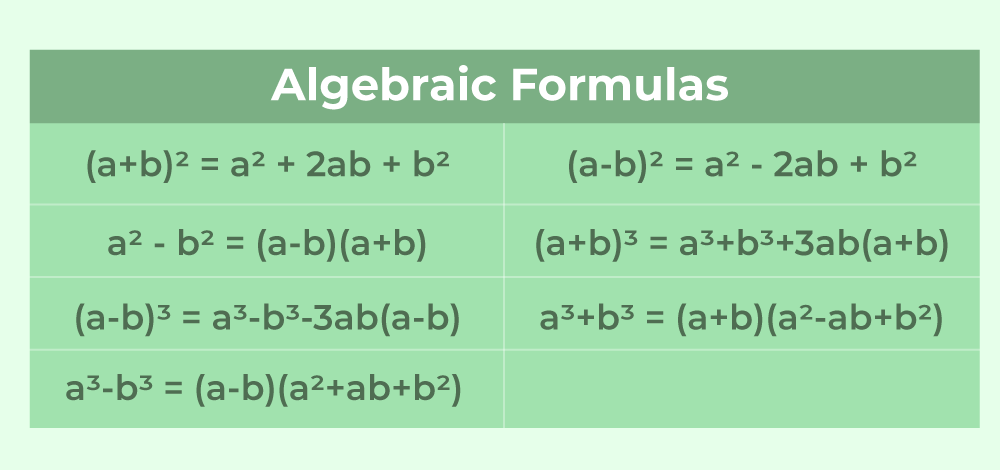### Geometry

Geometry is a part of mathematics that is concerned with the study of shapes, sizes, parameters, measurements, properties, and dimensions. There are generally three types of geometry. They are Euclidean geometry, Spherical geometry, and Hyperbolic geometry.

Basic Geometry Formula

We study Geometry formulas under two headings that are,

• 2-D Formulas
• 3-D Formulas

## 2-D Formulas

• Rectangle
• Perimeter of Rectangle = 2(l + b)
• Area of Rectangle  = l × b

Where
l’ is Length

• Square
• Area of Square = a2
• Perimeter of Square = 4a

Where
a’ is the length of sides of a Square

• Triangle
• Area of Triangle= 1/2 × b × h

Where
b’ is the base of the triangle and
h’ is the height of the triangle

• Trapezoid
• Area of Trapezoid = 1/2 × (b1 + b2) × h

Where
b1 and b2 are the bases of Trapezoid
h is height of Trapezoid

• Circle
• Area of Circle = π × r2
• Circumference of Circle = 2πr

Where
r’ is radius of a Circle

Various formulas used for 2-D objects are,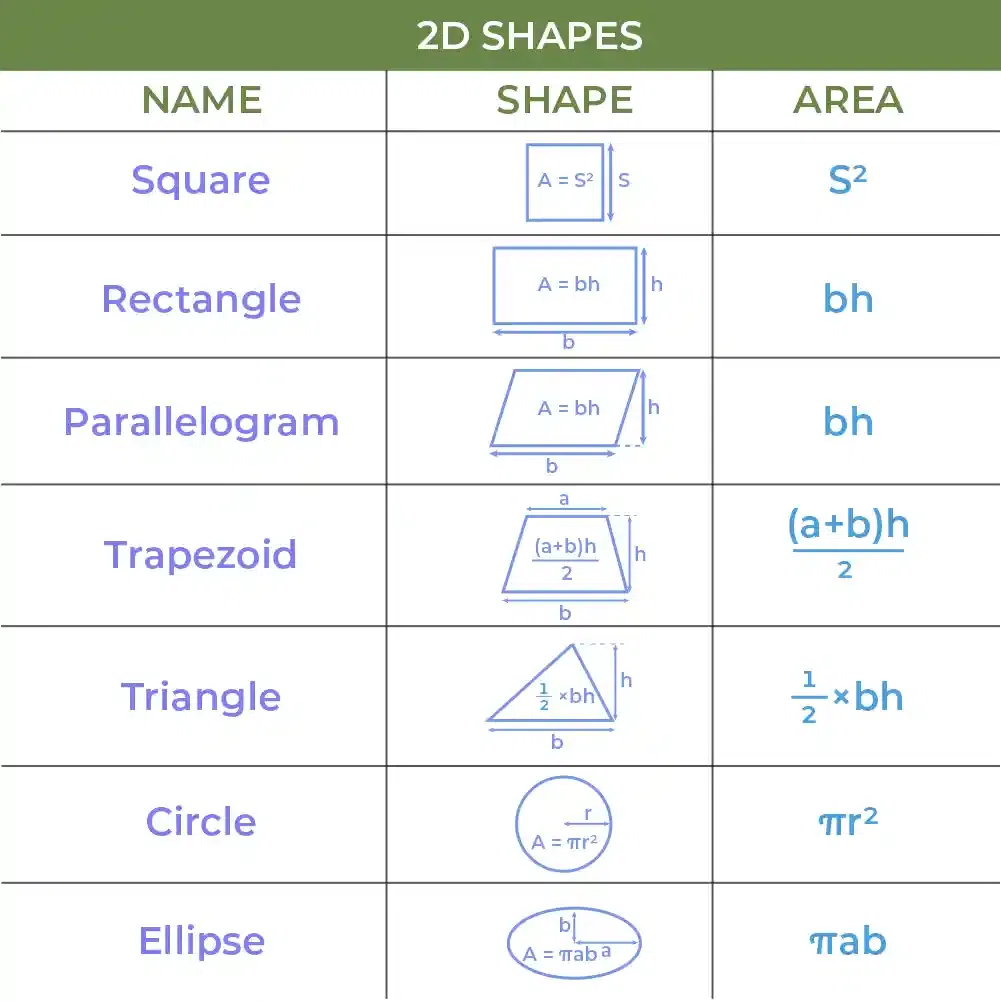## 3-D Formulas

• Cube
• Surface Area of Cube = 6a2
• Volume of Cube = a3

Where
a’ is the length of sides of Cube

• Cylinder
• Curved Surface Area of Cylinder = 2πrh
• Total Surface Area of Cylinder = 2πr(r + h)
• Volume of Cylinder = V = πr2h

Where
r’ is the radius of base of Cylinder
h’ is the height of Cylinder

• Cone
• Curved Surface Area of Cone = πrl
• Total Surface Area of Cone = πr(r + l) = πr[r + √(h2 + r2)]
• Volume of Cone = V = 1/3× πr2h

where,
r’ is the Radius of base of Cone
h is the Height of the Cone

• Sphere
1. Surface Area of a Sphere = S = 4πr2
2. Volume of a Sphere = V = 4/3 × πr3

Where,
r is the Radius of Sphere

Various formulas used for 3-D objects are,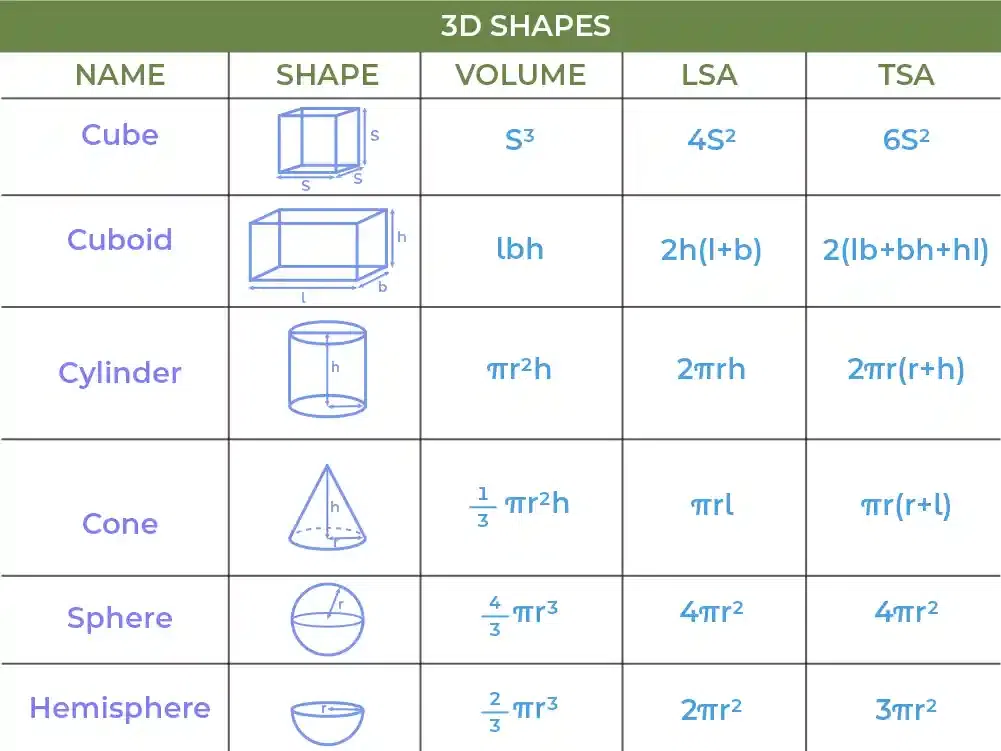### Probability

Probability is the mathematical term used to determine the chance of occurring a particular event. Probability can simply be defined as the possibility of the occurrence of an event. It is expressed on a linear scale from 0 to 1. There are three types of theoretical probability, experimental probability, and subjective probability.

Basic Probability Formula

P(A) = n(A)/n(S)

Where
P(A) is the Probability of an Event.
n(A) is the Number of Favourable Outcomes
n(S) is the Total Number of Events

### Fraction

A fraction is a number expressed with integers in which a numerator is divided by the denominator. A fraction is basically the quotient of a division.

Basic Fractions Formula

• (a + b/c) = [(a × c) + b]/c
• (a/b + d/b) = (a + d)/b
• (a/b + c/d) = (a × d + b × c)/(b × d)
• a/b × c/d = ac/bd
• (a/b)/(c/d) = a/b × d/c

### Percentage

A percentage is a numerical value or ratio expressed as a fraction of 100. It is generally symbolized by the sign %.

Basic Percentage Formula

Percentage = (Given Value/Total Value) × 100

### Distance Formula

If the coordinate of the points A is (x1, y1) and B is (x2, y2) the formula used to calculate the distance between these two points is discussed in the image below.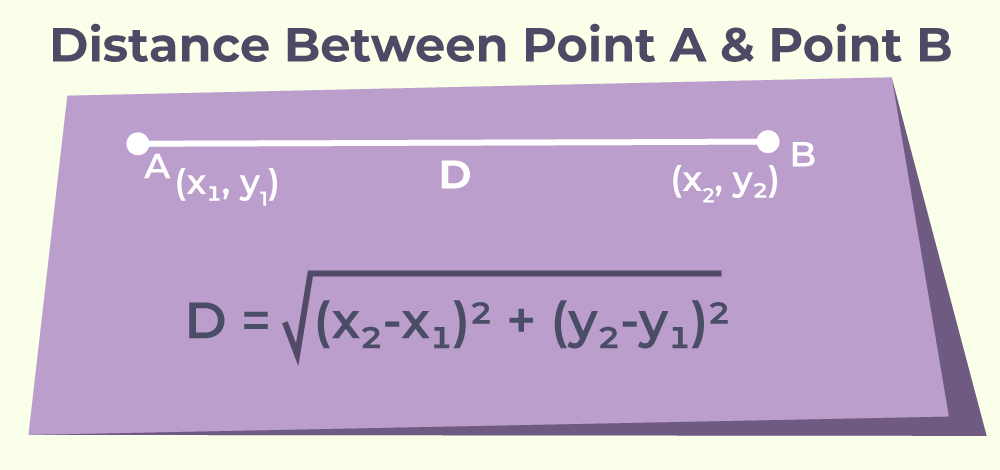### Trigonometry Formulas

There are six basic functions of Trigonometry are,

• SIn Function
• Cos Function
• Tan Function
• Cosec function
• Sec Function
• Cot Function

The three basic trigonometric functions formulas are,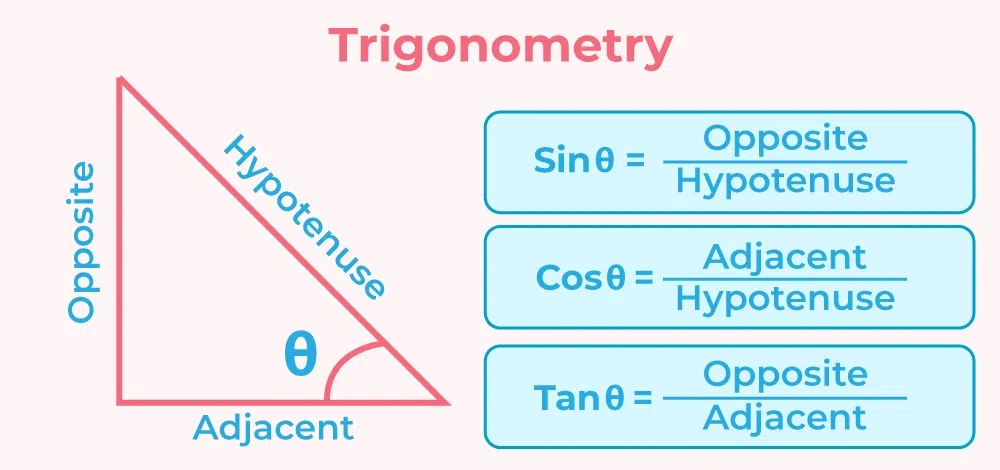## List of Math Formulas

The list of most used maths formulas is,

## Maths Formula Sheet

All the general formulas used in Mathematics are discussed below.

## Solved Examples on Math Formulas

Example 1: Determine the probability to get an ace from a card taken from a deck.

Solution:

Given:

Total number of favorable outcomes n(S) = 52

Number of face cards  in the deck = 12

Number of favorable outcomes n(A) = 12

Now,

P(A) = n(A)/n(S)

= 12/52

= 3/13

Hence, the probability to get a face card from a card deck is 3/13.

Example 2: Simplify 3/(x – 1) + 1/(x – 1) = 2/x

Solution:

3/(x-1) + 1/(x – 1) = 2/x

4/(x – 1) = 2/x

2x = x – 1

x = -1

Example 3: If x + 1/x = 3. Find the value of x2 + 1/x2.

Solution:

Given,

x + 1/x = 3

Squaring both side

x + 1/x)2 = (3)2

x2 + 2 × x × 1/x + (1/x)2 = 9

x2 + 1/x2 + 2 = 9

x2 + 1/x2 = 7

Example 4: If the radius of a circle is 21cm. Find the area of the given circle.

Solution:

Given:

We have,

Area of the circle (A) = πr2

A = 22/7 × 21 × 21

A = 1386cm2

Hence, the area of the given circle is 1386cm2

Example 5: Find the area of a triangle having a base of 100cm and a height of 20cm.

Solution:

Given:

Base of the triangle =100cm

Height of the triangle = 20cm

We have,

Area(A) = 1/2 × b × h

= 1/2 × 10 × 20

= 1000cm2

Example 6: Punam has 4/5 parts of the field among which she uses 2/5 parts for farming. How many parts of the farm are left for other purposes?

Solution:

Given,

Total Fraction of Land = 4/5

Total Fraction used for Farming = 2/5.

Now,

Land  Left = 4/5 – 2/5

= 2/5

Hence, 2/5 part of the field is left for other purposes.

Example 7: What will be the 20% of 240 kg?

Solution:

Given,

= 20% of 240kg

= 20/100 × 240

= 48 kg

Hence, 20% of 240 kg will be 48 kg.

## FAQs on Math Formulas

### Q1: What are some basic Math formulas?

Some basic Math formulas are :

1. Perimeter of square = 4×a
2. Perimeter of rectangle = 2 (l + b)
3. Circumference of circle = 2 (pi) r
4. Area of square = a2
5. Area of rectangle = l × b

### Q2: What is the formula of a3 + b3?

a3 + b3 = (a + b) (a2 – ab + b2)